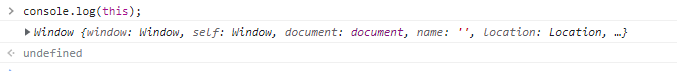# JavaScript 中 this 关键字的作用及改变其上下文的方法

JavaScript 中的 this 关键字引用了所在函数正在被调用时的对象。在不同的上下文中，this 的指向会发生变化。可以通过 call, apply, bind 方法来改变 this 的上下文。

## 一、this 关键字的作用

JavaScript 中的 `this` 关键字引用了所在函数正在被调用时的对象。在不同的上下文中，`this` 的指向会发生变化。```class MyClass {
constructor() {
this.value = 42;
}
}

let obj = new MyClass();
console.log(obj.value); // 42```

```class MyClass {
value = 42;
printValue() {
console.log(this.value);
}
static printValue() {
console.log(this.value);
}
}

let obj = new MyClass();
obj.printValue(); // 42
MyClass.printValue(); // undefined```

```let baseObject = { value: 42 };
let obj = Object.create(baseObject);

function printValue() {
console.log(this.value);
}

printValue.call(obj); // 42```

### 在类中使用箭头函数

```class MyClass {
value = 42;
printValue = () => {
console.log(this.value);
}
}
let obj = new MyClass();
obj.printValue(); // 42```

```class MyClass {
constructor() {
this.value = 42;
}
}

let obj = MyClass(); // without new keyword
console.log(obj); // undefined
console.log(value); // 42```

### 在对象的方法中使用箭头函数会导致 this 指向问题

```let obj = {
value: 42,
printValue: () => {
console.log(this.value);
}
};
obj.printValue(); // undefined```

```let obj = {
value: 42,
printValue: function(){
console.log(this.value);
}
};
obj.printValue(); // 42```

```let obj = {
value: 42,
printValue: () => {
console.log(obj.value);
}
};
obj.printValue(); // 42```

## 二、如何改变 this 上下文

`call``apply` 方法允许您将函数的 `this` 指向指定的对象，并立即执行该函数。

`call` 方法的语法格式如下：

`functionName.call(thisArg, arg1, arg2, ...);`

`apply` 方法的语法格式如下：

`functionName.apply(thisArg, [arg1, arg2, ...]);`

`bind` 方法允许您将函数的 `this` 指向指定的对象，但不立即执行函数，而是返回一个新函数，可以在将来执行。

`let newFunc = functionName.bind(thisArg, arg1, arg2, ...);`

```let obj = {value: 42};

function printValue() {
console.log(this.value);
}

printValue.call(obj); // 42
printValue.apply(obj); // 42
let boundFunc = printValue.bind(obj);
boundFunc(); // 42```

0
0

0 条回复（回复会通过微信通知作者）

（微信扫码即可登录，无需注册）